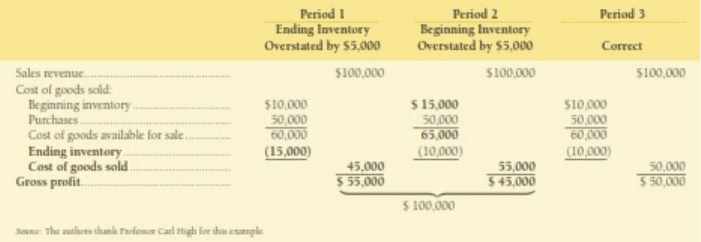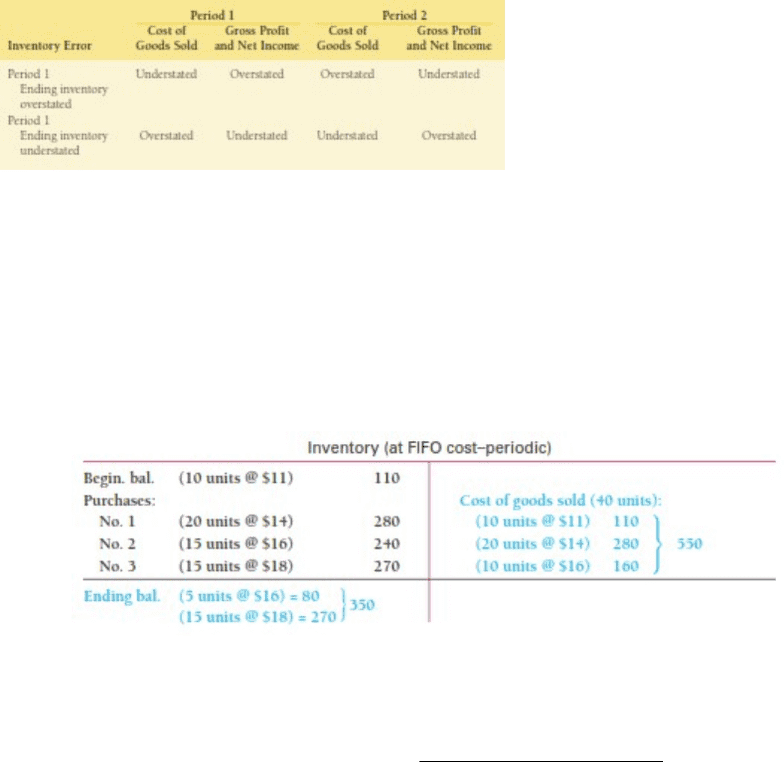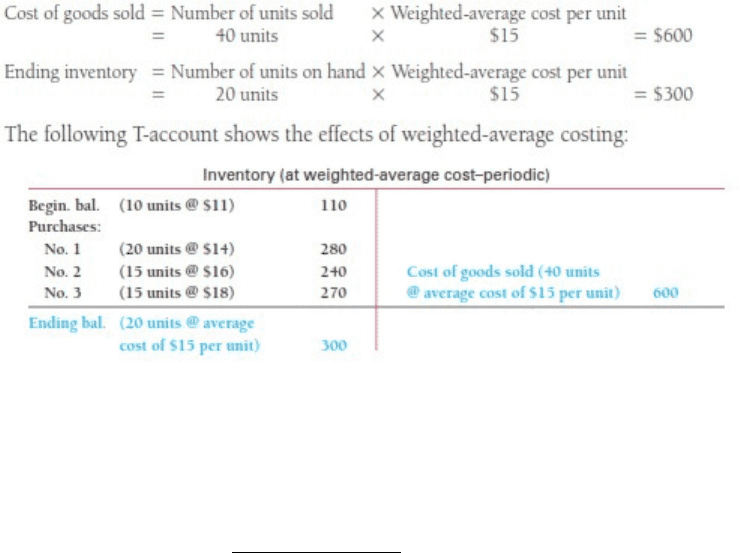Study Guides (400,000)
CA (150,000)
McMaster (9,000)
COMMERCE (1,000)
Final

# COMMERCE 1AA3 Study Guide - Final Guide: Time Point, Intangible Asset, Effective Interest Rate

Department
Commerce
Course Code
COMMERCE 1AA3
Professor
Study Guide
Final

This preview shows pages 1-3. to view the full 26 pages of the document.Chapter 6: Inventory and Cost of Goods Sold
When there are errors when counting inventory, there are various overstatements and
understatements:
oWhen ending inventory in period 1 is overstated by
X
, cost of goods sold is
then understated by
X
. This, in turn, makes gross profit overstated by
X
.
This error carries over in period 2, where beginning inventory is now overstated
by
X
. The cost of goods available for sale is then overstated by
X
. This, in
turn, understates gross profit by
X
. Income tax is also understated due to the
understatement in income. The errors in two consecutive periods counteract one
another. Thus, in period three, all errors are corrected due to the
counterbalancing effect.
o
oSimilarly, understating ending balance of inventory by
X
in period one also
overstates costs of goods sold by
X
. This understates gross profit by
X
.
In period 2, the beginning balance of inventory is now understated by
X
. This
makes the cost of goods available for sale also understated by
X
. This, in
turn, overstates gross profit by
X
. Income tax is also overstated due to the
overstatement in income. The errors in two consecutive periods counteract one
another. Thus, in period three, all errors are corrected due to the
counterbalancing effect.
oNote that ending inventory and gross profit have a direct relationship.
oAlso note that beginning inventory and gross profit have an inverse relationship.

Only pages 1-3 are available for preview. Some parts have been intentionally blurred.o
There are two important methods when inventory costing:
oFirst-in, first-out (FIFO, periodic method)
This method assumes that the beginning balance of inventory and the
initial purchases of inventory are sold before the inventory purchased
later in the period
oWeighted-average cost (Periodic method)
Weightedaveragecost per unit =cost of goodsavailable
number of units available
Note that
cost of goods available=beginning inventory+purchases

Only pages 1-3 are available for preview. Some parts have been intentionally blurred.Gross profit/margin percentage is a ratio that measures the percent of each dollar that
is considered profit. The remaining percent is the consumption of cost of goods sold.
This percentage is calculated by the equation
o
Gross profit percentage=Gross profit
Net sales revenue
, where
gross profit=COGASEBI
oIf, for example, the gross profit percentage is
, each dollar of sales
generates 45 cents of profit and cost of goods sold consumes 55 cents.
oWhen estimating ending inventory using this percentage, the following steps are
followed
First, note that
endinginventory =beginning balance+purchasescost of goodssold
where
beginning balance+purchases=cost of goods available for sale
cost of goods sold
is an estimate that uses previous net sales revenue
and subtracting the gross-profit margin percent of net sales revenue
This process is illustrated in the following example